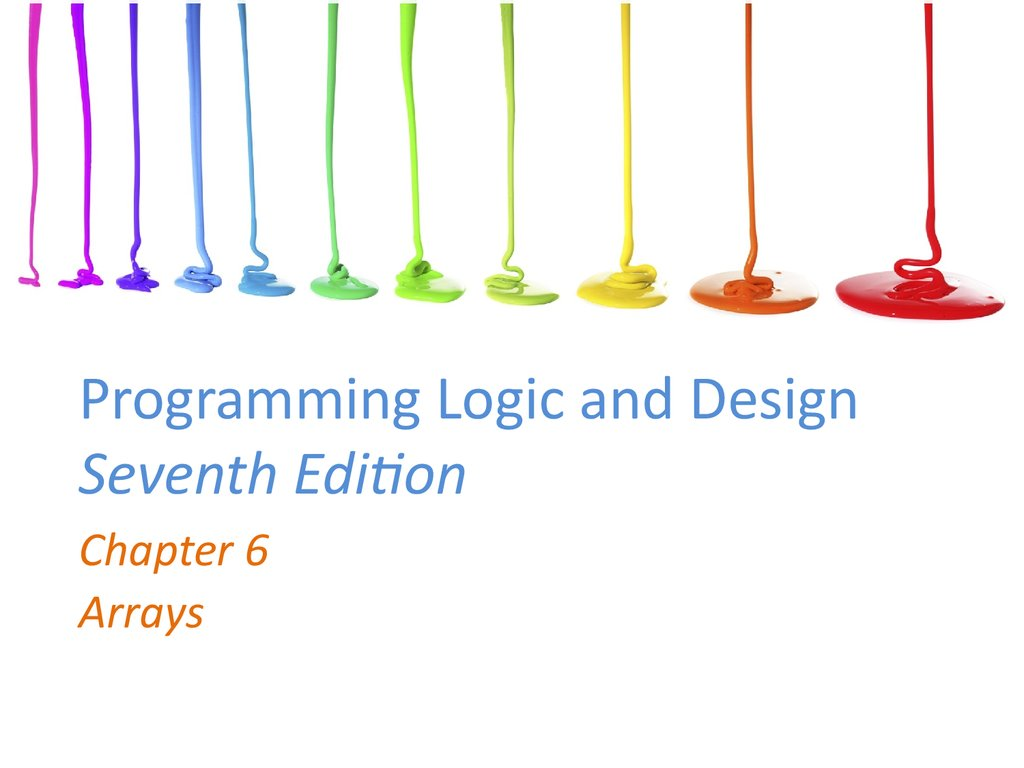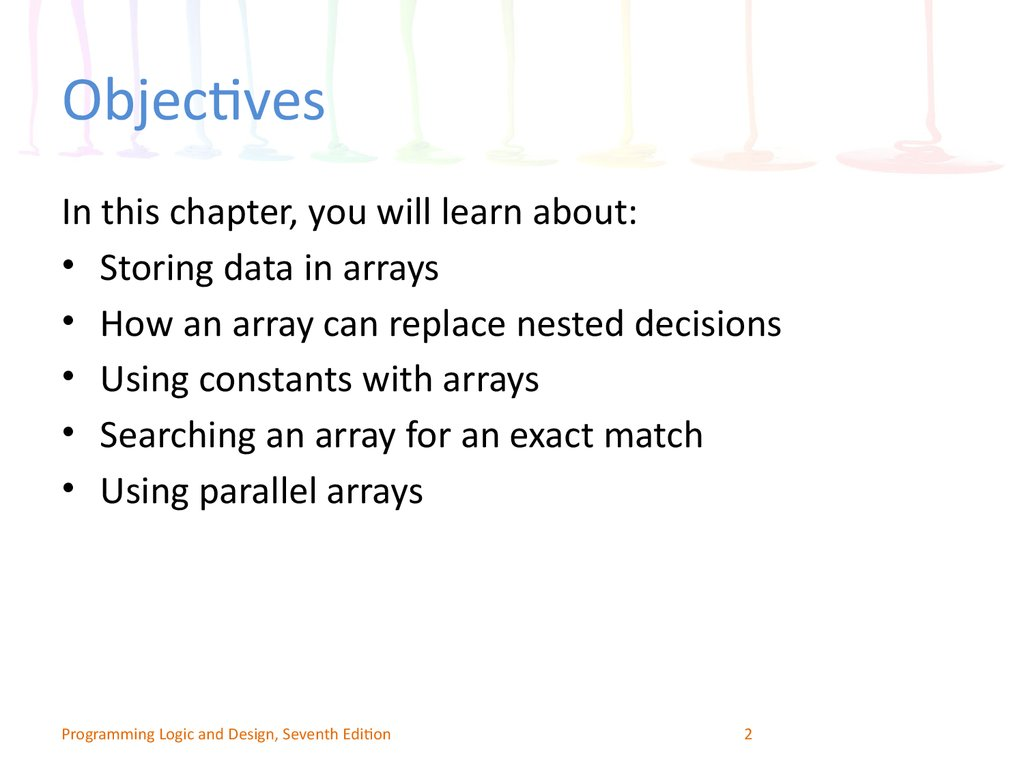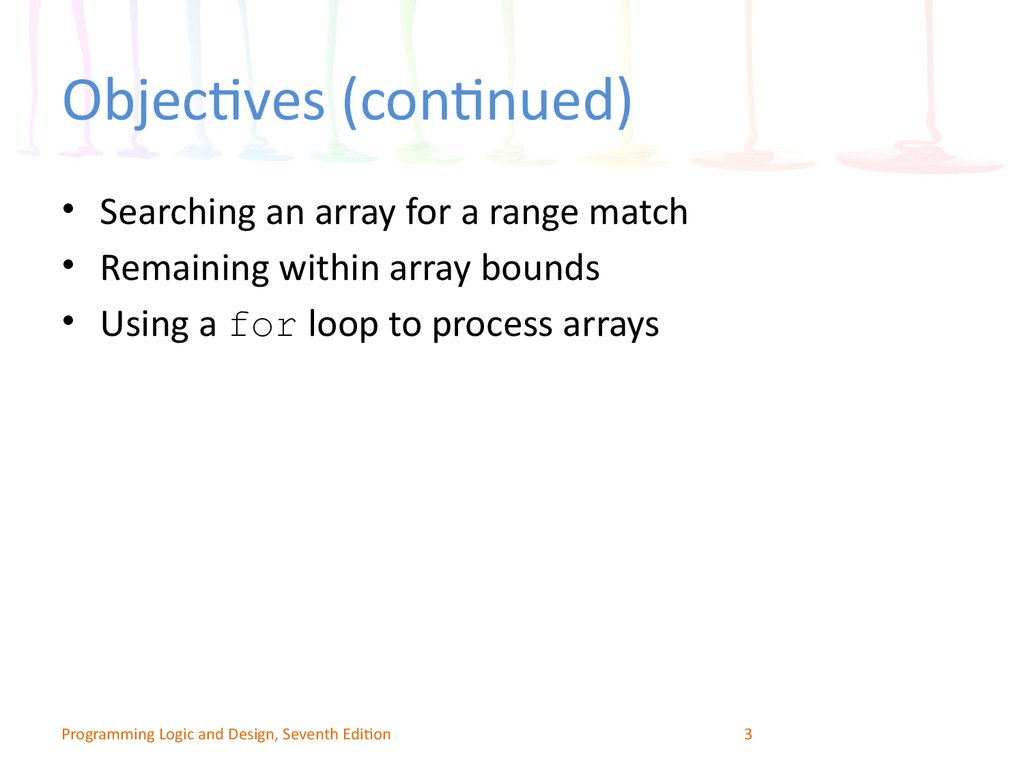Programming Logic and Design Seventh Edition

Chapter 6
Arrays

2. Objectives

In this chapter, you will learn about:
• Storing data in arrays
• How an array can replace nested decisions
• Using constants with arrays
• Searching an array for an exact match
• Using parallel arrays
Programming Logic and Design, Seventh Edition
2

3. Objectives (continued)

• Searching an array for a range match
• Remaining within array bounds
• Using a for loop to process arrays
Programming Logic and Design, Seventh Edition
3

4. How Arrays Occupy Computer Memory

• Array
– A series or list of variables in computer memory
– All variables share the same name
– Each variable has a different subscript
• Subscript (or index)
– Position number of an item in an array
– Subscripts are always a sequence of integers
Programming Logic and Design, Seventh Edition
4

5.

How Arrays Occupy Computer
Memory (continued)
• Each item has the same name and the same data type
• Element: an item in the array
– Array elements are contiguous in memory
• Size of the array: the number of elements it will hold
• Subscripts or indexes: indicate the position of a
particular element in the array
• Adding data values is called populating the array
Programming Logic and Design, Seventh Edition
5

6. How Arrays Occupy Computer Memory (continued)

Figure 6-1 Appearance of a three-element array in computer memory
Programming Logic and Design, Seventh Edition
6

7. How an Array Can Replace Nested Decisions

• Example: Human Resources Department Dependents
report
– List employees who have claimed zero through five
dependents
• Assume no employee has more than five dependents
• Application produces counts for dependent
categories
– Uses a series of decisions
• Application does not scale up to more dependents
Programming Logic and Design, Seventh Edition
7

8.

Figure 6-3 Flowchart and pseudocode of decision-making process using a series
of decisions—the hard way
Programming Logic and Design, Seventh Edition
8

9. How an Array Can Replace Nested Decisions (continued)

• The array reduces the number of statements needed
• Six dependent count accumulators are redefined as a
single array
• Variable as a subscript to the array
• Array subscript variable must be:
– Numeric with no decimal places
– Initialized to 0
– Incremented by 1 each time the logic passes through the
loop
Programming Logic and Design, Seventh Edition
9

10.

Figure 6-4 Flowchart and pseudocode of decision-making process—but still the hard way
Programming Logic and Design, Seventh Edition
10

11.

Figure 6-5 Flowchart and pseudocode of decision-making process
using an array—but still a hard way
Programming Logic and Design, Seventh Edition
11

12. How an Array Can Replace Nested Decisions (continued)

Figure 6-6 Flowchart and pseudocode of efficient decision-making
process using an array
Programming Logic and Design, Seventh Edition
12

13.

Figure 6-7 Flowchart and pseudocode for Dependents report program
Programming Logic and Design, Seventh Edition
13

14. How an Array Can Replace Nested Decisions (continued)

Figure 6-7 Flowchart and pseudocode for Dependents report program (continued)
Programming Logic and Design, Seventh Edition
14

15. Using Constants with Arrays

• Use constants in several ways
– To hold the size of an array
– As the array element values
– As an array subscript
Programming Logic and Design, Seventh Edition
15

16. Using a Constant as the Size of an Array

• Avoid “magic numbers” (unnamed constants)
• Declare a named numeric constant to be used every
time the array is accessed
• Make sure any subscript remains less than the
constant value
• Constants are created automatically in many
languages
Programming Logic and Design, Seventh Edition
16

17. Using Constants as Array Element Values

• Sometimes the values stored in arrays should be
constants
• Example
string MONTH = "January",
"February", "March", "April", "May",
"June", "July", "August", "September",
"October", "November", "December"
Programming Logic and Design, Seventh Edition
17

18. Using a Constant as an Array Subscript

• Use a numeric constant as a subscript to an array
• Example
– Declare a named constant as: num INDIANA = 5
– Display value with:
output salesArray[INDIANA]
Programming Logic and Design, Seventh Edition
18

19. Searching an Array for an Exact Match

• Sometimes you must search through an entire array to
find a value

Item numbers are three-digit, non-consecutive numbers
Customer orders an item; check if item number is valid
Create an array that holds valid item numbers
Search the array for an exact match
Programming Logic and Design, Seventh Edition
19

20.

Figure 6-8 Flowchart and pseudocode for a program that verifies item availability
Programming Logic and Design, Seventh Edition
20

21.

Figure 6-8 Flowchart and pseudocode for a program that verifies item availability (continued)
Programming Logic and Design, Seventh Edition
21

22.

Figure 6-8 Flowchart and pseudocode for a program that verifies item availability (continued)
Programming Logic and Design, Seventh Edition
22

23. Searching an Array for an Exact Match (continued)

• Flag: a variable that indicates whether an event
occurred
• Technique for searching an array
– Set a subscript variable to 0 to start at the first element
– Initialize a flag variable to false to indicate the desired value
has not been found
– Examine each element in the array
– If the value matches, set the flag to True
– If the value does not match, increment the subscript and
examine the next array element
Programming Logic and Design, Seventh Edition
23

24. Using Parallel Arrays

– Two arrays, each with six elements
• Valid item numbers
• Valid item prices
– Each price in the valid item price array is in the same position as
the corresponding item in the valid item number array
• Parallel arrays
– Each element in one array is associated with an element in the
same relative position in the other array
• Look through the valid item array for the customer’s item
– When a match is found, get the price from the item price array
Programming Logic and Design, Seventh Edition
24

25. Using Parallel Arrays (continued)

Figure 6-9 Parallel arrays in memory
Programming Logic and Design, Seventh Edition
25

26. Using Parallel Arrays (continued)

• Use parallel arrays
– Two or more arrays contain related data
– A subscript relates the arrays
• Elements at the same position in each array are logically related
• Indirect relationship
– Relationship between an item’s number and its price
– Parallel arrays are very useful
Programming Logic and Design, Seventh Edition
26

27.

Figure 6-10 Flowchart and pseudocode of a program that finds an item price using
parallel arrays
Programming Logic and Design, Seventh Edition
27

28.

Figure 6-10 Flowchart and pseudocode of a program that finds an item price using
parallel arrays (continued)
Programming Logic and Design, Seventh Edition
28

29.

Figure 6-10 Flowchart and pseudocode of a program that finds an item price using
parallel arrays (continued)
Programming Logic and Design, Seventh Edition
29

30. Improving Search Efficiency

• The program should stop searching the array when
a match is found
• Set a variable to a specific value instead of letting
normal processing set it
• Improves efficiency
• The larger the array, the better the improvement by
doing an early exit
Programming Logic and Design, Seventh Edition
30

31.

Figure 6-11 Flowchart and pseudocode of the module that finds an item price and
exits the loop as soon as it is found
Programming Logic and Design, Seventh Edition
31

32. Improving Search Efficiency (continued)

Figure 6-11 Flowchart and pseudocode of the module that finds an item price and
exits the loop as soon as it is found (continued)
Programming Logic and Design, Seventh Edition
32

33. Searching an Array for a Range Match

• Programmers may want to work with ranges of
values in arrays, 1 through 5 or 20 through 30
– Read the customer order data; determine the discount
based on the quantity ordered
• First approach
– An array with as many elements as each possible order
quantity
– Store the appropriate discount for each possible order
quantity
Programming Logic and Design, Seventh Edition
33

34. Searching an Array for a Range Match (continued)

Figure 6-13 Usable—but inefficient—discount array
Programming Logic and Design, Seventh Edition
34

35. Searching an Array for a Range Match (continued)

• Drawbacks of first approach
– Requires a very large array; uses a lot of memory
– Stores the same value repeatedly
– How do you know when you have enough elements?
• Customer can always order more
• Better approach
– Create four discount array elements for each discount
rate
– A parallel array with a discount range
• Use a loop to make comparisons
Programming Logic and Design, Seventh Edition
35

36. Searching an Array for a Range Match (continued)

Figure 6-14 Parallel arrays to use for determining discount
Programming Logic and Design, Seventh Edition
36

37.

Figure 6-15 Program that determines discount rate
Programming Logic and Design, Seventh Edition
37

38. Remaining within Array Bounds

• Every array has a finite size
– Number of elements in the array
– Number of bytes in the array
• Arrays are composed of elements of the same data type
• Elements of the same data type occupy the same
number of bytes in memory
• The number of bytes in an array is always a multiple of
the number of array elements
• Access data using a subscript containing a value that
accesses memory occupied by the array
Programming Logic and Design, Seventh Edition
38

39. Remaining within Array Bounds (continued)

Figure 6-16 Determining the month string from user’s numeric entry
Programming Logic and Design, Seventh Edition
39

40. Remaining within Array Bounds (continued)

• Program logic assumes every number entered by
the user is valid
• When an invalid subscript is used:
– Some languages stop execution and issue an error
– Other languages access a memory location outside of the
array
• An invalid array subscript is a logical error
• Out of bounds: using a subscript that is not within
the acceptable range for the array
• The program should prevent bounds errors
Programming Logic and Design, Seventh Edition
40

41. Using a for Loop to Process Arrays

• for loop: a single statement
– Initializes the loop control variable
– Compares it to a limit
– Alters it
• The for loop is especially convenient when
working with arrays
– To process every element
• Must stay within array bounds
• Highest usable subscript is one less than the array
size
Programming Logic and Design, Seventh Edition
41

42. Using a for Loop to Process Arrays (continued)

Figure 6-17 Pseudocode that uses a for loop to display an array of department names
Programming Logic and Design, Seventh Edition
42

43. Using a for Loop to Process Arrays (continued)

Figure 6-18 Pseudocode that uses a more efficient for loop to output department names
Programming Logic and Design, Seventh Edition
43

44. Summary

• Array: a named series or list of values in memory
– Same data type
– Different subscript
• Use a variable as a subscript to the array to replace
multiple nested decisions
• Constants can be used to hold an array’s size
• Searching through an array requires
– Initializing a subscript
– Using a loop to test each element
– Setting a flag when a match is found
Programming Logic and Design, Seventh Edition
44

45. Summary (continued)

• Parallel arrays: each element in one array is
associated with the element in a second array
– Elements have the same relative position
• For range comparisons, store either the low- or
high-end value of each range
Programming Logic and Design, Seventh Edition
45

46. Summary (continued)

• Access data in an array
– Use a subscript containing a value that accesses memory
occupied by the array
• A subscript is out of bounds if it is not within the
defined range of acceptable subscripts
• The for loop is a convenient tool for working with
arrays
– Process each element of an array from beginning to end
Programming Logic and Design, Seventh Edition
46